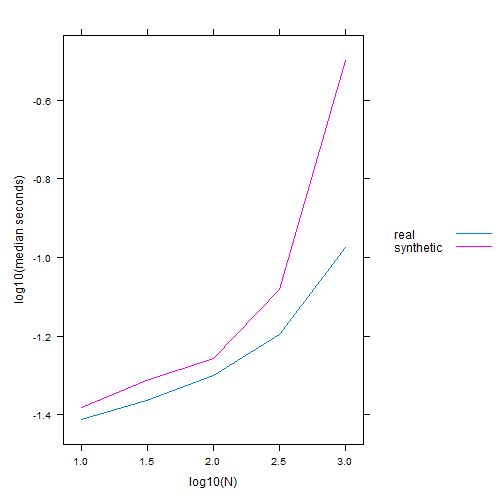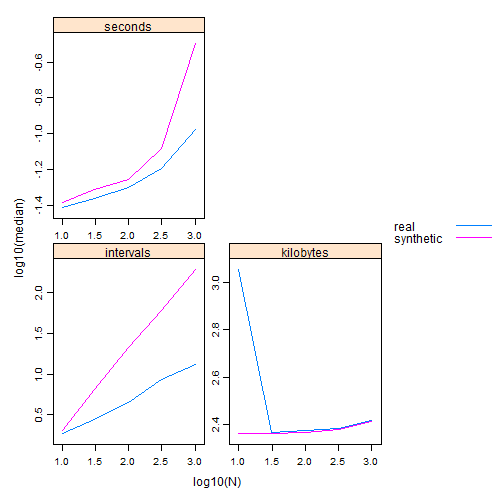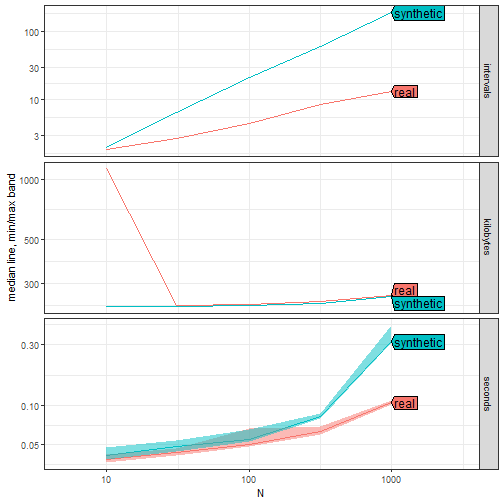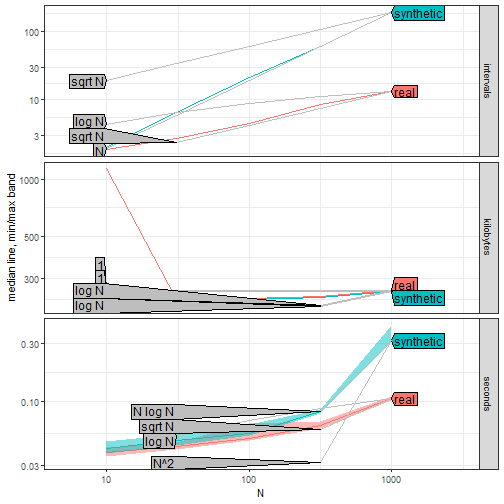The time complexity of the Dynamic Programming algorithm implemented in the PeakSegDisk package depends on the number of intervals (candidate changepoints stored). Here we compute the mean number of intervals for real Mono27ac data, and synthetic count data which are always increasing.

``````old.opt <- options(width=100)
data(Mono27ac, package="PeakSegDisk", envir=environment())
library(data.table)
penalty <- 1e6
atime.list <- atime::atime(
N=as.integer(10^seq(1, 3, by=0.5)),
setup={
some.cov <- Mono27ac\$coverage[1:N]
some.inc <- data.table(some.cov)
some.inc[, count := 1:.N]
},
seconds.limit=Inf,
real={
PeakSegDisk::PeakSegFPOP_df(some.cov, penalty)
},
synthetic={
PeakSegDisk::PeakSegFPOP_df(some.inc, penalty)
})
plot(atime.list)
``````The plot above shows the timings in both kinds of data. Clearly the algorithm is much faster in real data than in synthetic increasing data. The code below creates a new column for the mean number of intervals computing during the algorithm, then computes the best asymptotic references:

``````atime.list\$measurements[, intervals := sapply(result, function(L)L\$loss\$mean.intervals)]
best.list <- atime::references_best(atime.list, more.units="intervals")
plot(best.list)
``````Note in the code above the `more.units="intervals"` argument, which says to use the intervals column as an additional unit. The plot above shows plots of all three units as a function of data size. It is clear that there is a substantial difference in the number of intervals stored by the algorithm, between real and synthetic increasing data. Next we plot the same data using a different method.

``````if(require(ggplot2)){
gg <- ggplot()+
theme_bw()+
facet_grid(unit ~ ., scales="free")+
geom_line(aes(
N, empirical, color=expr.name),
data=best.list\$meas)+
geom_ribbon(aes(
N, ymin=min, ymax=max, fill=expr.name),
data=best.list\$meas[unit=="seconds"],
alpha=0.5)+
scale_x_log10()+
scale_y_log10("median line, min/max band")
(gg.show <- if(require(directlabels)){
gg+
directlabels::geom_dl(aes(
N, empirical, color=expr.name, label=expr.name),
method="right.polygons",
data=best.list\$meas)+
theme(legend.position="none")+
coord_cartesian(xlim=c(5,3000))
}else{
gg
})
}
``````Note in the plot above that there are error bands for the seconds panel, and direct labels instead of a legend. The code below augments this plot by adding two asymptotic references for each empirical curve (next closest under and over).

``````best.ref <- best.list\$ref[each.sign.rank==1]
if(require(ggplot2)){
gg.ref <- gg.show+
geom_line(aes(
N, reference, group=paste(fun.name, expr.name)),
color="grey",
data=best.ref)
if(require(directlabels)){
gg.ref+
directlabels::geom_dl(aes(
N, reference,
label.group=paste(fun.name, expr.name),
label=fun.name),
data=best.ref,
color="grey",
method="left.polygons")
}else{
gg.ref
}
}
``````From the plot above it is clear that

• the number of intervals grows slowly (log) for real data, and much faster (linear) for synthetic increasing data,
• the memory usage (kilobytes) is grows slowly (log or constant),
• the computation time grows slowly for real data (expected log-linear), and much faster for synthetic increasing data (expected quadratic).

Exercise for the reader: to see the expected asymptotic time complexity in the last plot, re-do the previous analyses, increasing the penalty as well as the max data size N.

``````options(old.opt)
``````